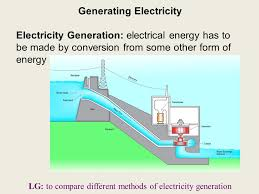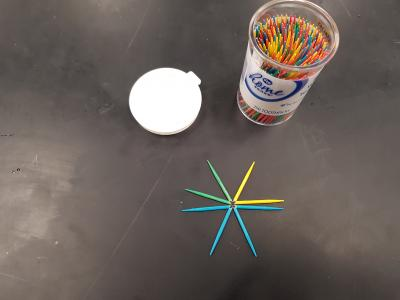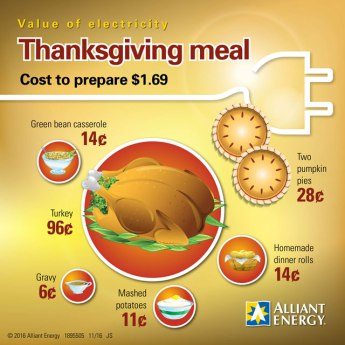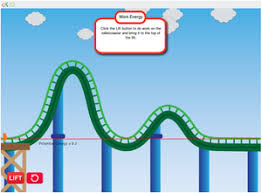Se muestran los artículos pertenecientes a Noviembre de 2017.

## 7th GRADE SCIENCE : Article 23 : Energy sources.* Activity 2.9   :   Water power. Electricity generation.                              ( 11/6/17   Monday )

HYDRO (WATER) POWER.

questions given to you today.
( 10 points ).

following questios.  You can do it thoughout using a google docs.

a) What is the force used in an hydroplant in order to generate
electricity?.

b)  The turbine generates electricity as it is hit by running
water at a high speed. Why then are TRANSFORMERS
needed before sending electricity to the customers?.

c)   Why is important to be able to produce electricity from
these hydroplants in order to help conserve our
environment?.
( 10 points ).

2.9.c                     You may watch the following  videos so that you can understand

better how electricity is generated.

Video 1

* Activity 2.10   :   Potential energy into Kinetic energy.                            ( 11/7/17   Tuesday )

2.10.a               Look up the followings links :

and afterwards answer the following questions and exercises.

( 10 points ).

2.10.b        Look up the followings links and explain in a google docs how
potential energy is transformed or transferred into kinetic energy.

( 10 points ).

* Activity 2.11   :   Geothermal energy.                                                  ( 11/8/17   Wednesday )

GEOTHERMAL ENERGY.

2.11.a               Understanding what geothermal energy is.

a) What the geothermal energy is?.

b) Where does it come from?.

c) Explain the path geothermal energy follows until is used
on the earths surface.

d)  What does water have to do with this geothermal energy?.

e)  Which states in USA is most geothermal energy being used?.

( 10 points ).

2.11.b              Is geothermal energy a renewable one? :

a) Look the same link than before and explain why geothermal
energy is considered as a renewable kind of energy source.

( 5 points ).

2.11.c              Draw the different parts a geothermal energy installation has  :

On a piece of paper make an outline about a geothermal
plant indicating its different parts.

( 5 points ).

* Activity 2.12   :  Solar  energy.                                                              ( 11/9/17   Thursday )

SOLAR ENERGY.

2.12.a                Today you work in groups of three people. Each one has to prepare
only one solar energy sources different parts. You decide and agree
among the groups members which part you choose.
Each person must make an outline about his part and tell it to the
others. When everybody has finished his oral presentation
everyone has to write a summarize about the whole issue including the
others parts, in order to learn the general idea about the whole solar
energy system.

Person 1 :
solar energy consists of, what it is and which  the main
important features are in order to develop it. When you finish

Person 2 :
solar is found.
When you finish your writing, read out it loud to the others.

AND SOLAR THERMAL POWER PLANTS.

Person 3 :
solar energy consists of, what it is and which  the main
important features are in order to develop it. When you finish

SOLAR THERMAL COLLECTORS AND ENVIRONMENT.

( 15 points ).

2.12.b       When you hear about all the others parts, make a report about the whole
you have listened to and email it to me reporting the most important you
have written down about the three parts of the solar energy source.

( 10 points ).

* Activity 2.13   :   Wind power and energy.                                                ( 11/10/17   Friday )

WIND POWER AND ENERGY.

Answer these questions in a google docs file and share it to me.

a) What is the wind?. Explain what it is.

b) Why does wind have so much energy?.

c) How do we take advantage of the Winds energy?.

d) Why are Wind turbines usually sited on high hills and mountains?.

e)  What were Winds energy and forces used in the past for?.

f)  Why is Wind power considered as a renewable energy source?.

g) How much energy is produced in USA by Wind power?.

h) How many houses can be powered up to electricity by using only
one wind turbine?.

i)  To end, draw this outline about how winds are formed.
Do it on a piece of paper.

( 15 points ).

2.13.b          Follow the instructions given in this video to make your pinwheel.

( 10 points )

## 7th GRADE SCIENCE : Article 24 : Potencial energy.* Activity 2.14   :   Elastic potencial energy.                                             ( 11/13/17   Monday )

ELASTIC POTENCIAL ENERGY.

2.14.a        Toothpicks elastic potencial energy.

and watch out for whats happening.     Notice the aftermath and answer
these questions ( use a piece of paper or a google docs ) :

1)  What has happended to the toothpicks once you added the water?.

2)  How would you explain what has happened?. What do you think about?.

3)  Which way do you think potencial energy has been involved in?.

4)  Where do you think potencial energy was stored in before adding water?.

( 15 points ).

2.14.b        Potencial energy.

5)  What is potencial energy?.

6)  What is then elastic potencial energy?.

7)  Would you change your answers to the some of the previous questions
asked at the activity 2.14.a ?.

8)  What do you think is the difference between the potencial energy involved
in toothpicks activity and the potencial energy involved when you let
drop a ball to the ground?.

9)  Either we consider elastic potencial energy or potencial energy, both
can end and be transformed in a different kind of energy. What is it?.

( 10 points ).

* Activity 2.15   :   Energy, forces and motion.                                             ( 11/14/17   Tuesday )

2.15.a    Transferring potential energy into kinetic energy throughout centrifugal force.

Follow the instructions given by your teacher in order to set up
the device using the Straw, the balloon, tape and paper clip.

When you have it ready, watch out what happens when you blow
it up. After finishing, answer the following questions.

( 10 points ).

2.15.b   When finished the experience, answer the following questions :

a) Which is the energy you used to make the device move?.

b) After blowing the balloon, what is the energy obtained when moving?.

c)  What is the force used for blowing the balloon?.

d)  What kind of force is making the device spin when air withdraws from
the balloon?.

e)  How many kind of energies and forces are involved in this experience?.
Name them.

( 10 points ).

* Activity 2.16   :   Balloon spinner questions .                                       ( 11/15/17   Wednesday )

2.16.a   Do and answer the following questions  yesterday you couldnt do :

a) Which is the energy you used to make the device move?.

b) After blowing the balloon, what is the energy obtained when moving?.

c)  What is the force used for blowing the balloon?.

d)  What kind of force is making the device spin when air withdraws from
the balloon?.

e)  How many kind of energies and forces are involved in this experience?.
Name them.

( 10 points ).

a) What does gravity have to do with the potential energy?.

b) In what direction is always going gravity?.

c) Do all the things fall at the same speed?

Why?   Who demonstrated that?.

d) Why was the Pissa tower important to demostrate gravity and the
same speed all objects fall down to earth?.

e) Who was Galileo?.

f)  What GPE means?

Explain what you think GPE is with your own words.

g) How many kinds of potential energy can we have?   Check it here !!

Summarize the most important of each of them.

i) Draw the Pissa tower and two objects falling at the same time just
as Galileo did in his experiment.

( 10 points ).

2.16.e        >  Here you have a brief outline about what potential energy is :

Potential Energy is the stored energy of an object given its position relative to a body. Gravitational potential energy is one type of potential energy and is equal to the product of the objects mass (m), the acceleration caused by gravity (g), and the objects height (h) as distance from the surface of the ground (the body).GPE = 3kg * 9.8m/s2 * 5m = 490J       (490 Joules ).

* Activity 2.17   :   Reviewing and working out potential energy .            ( 11/16/17   Thursday )

2.17.a    Read and check the following information. After that, use the formula to
work out the potential energy at the following wordings: (use a google docs).

1)  A stone falling from a building 250m high.

2)  A child of 50kg falling after jumping up until 0.6m high.

3)  A beisball falling after being thrown from 25m high.

Potential Energy is the stored energy of an object given its position relative to a body. Gravitational potential energy is one type of potential energy and is equal to the product of the objects mass (m), the acceleration caused by gravity (g), and the objects height (h) as distance from the surface of the ground (the body).GPE = 3kg * 9.8m/s2 * 5m = 490J       (490 Joules ).

( 5 points ).

2.17.b    Do the papers and word search given to you today.

Paper 1

Paper 2

Paper 3   ( Word search ).

( 10 points ).

* Activity 2.18   :   Potential and kinetic energies' Test .                           ( 11/17/17   Friday )

2.18.a   Open a google docs and copy  these questions and answer them.

Choose the best answer to each of the following questions. Good luck!
1. Niagara Falls is a good example of :
a. Kinetic energy being transformed into potential energy
b. Potential energy being transformed into kinetic energy
c. Energy being lost
d. Energy being created.
2. When energy moves from one form to another it is said to be _______________________.
a. Transfixed
b. Transformed
c. Eliminated
d. Lost.
.

3. On a day when the air is still a windmill still possesses
a. potential energy
b. kinetic energy
c. chemical energy
d. gravitational energy

4. The energy associated with motion is called
a. Kinetic energy
b. Elastic potential energy
c. Gravitational potential energy
d. Nuclear.

5.Moving water can be used to produce electricity because
a. Most forms of energy can be converted into other forms.
b. Energy cannot be converted into other forms.
c. Potential energy can be converted into kinetic energy, but not vice
versa.
d. Kinetic energy can be converted into potential energy, but not vice
versa.

6. Which of the following has kinetic energy?.

a. A rock poised for a fall
b. An archers bow that is drawn back
c. A rolling bowling ball
d. A car waiting at a red light

7. Unlike kinetic energy, potential energy is
a. Energy of motion
b. Stored
c. Conserved
d. Not measurable
8. Potential energy that depends on height is called
a. Kinetic energy
b. Gravitational potential energy
c. Elastic potential energy
d. Mechanical energy
9. What type of energy does a spinning turbine have?.

a. Electrical energy.
b. Nuclear energy.
c. Thermal energy.
d. Kinetic energy.

10. What is energy?

A. The ability to react chemically.
B. Anything on the periodic table.
C. The ability to change matter.
D. Only things that are moving have energy.

11. How are potential energy and kinetic energy different?

A. Potential energy is stored energy and kinetic energy is energy of motion.
B. Potential energy is energy of motion and kinetic energy is stored energy.
C. Potential energy is thermal energy and kinetic energy is radiant energy.
D. Potential energy is gravity and kinetic energy is chemistry.

12. Imagine a man bowling. In this case the bowling ball has

A. Potential
B. Kinetic
D. Nuclear                      ( 10 points ).

2.18.b     Go to this LINK, make play and do the  QUIZ .

( 10 points ).

2.18.c   Look up the questions at the following link. Copy them and

( 10 points ).

## 7th GRADE SCIENCE : Article 25 : THURSDAY 11/16/17 , Tasks to do.

* Activity 2.17   :   Reviewing and working out potential energy .            ( 11/16/17   Thursday )

2.17.a    Do the papers and word search given to you today.

Paper 1

Paper 2

Paper 3   ( Word search ).

( 10 points ).

2.17.b    Read and check the following information. After that, use the formula to
work out the potential energy at the following wordings: (use a google docs).

1)  A stone falling from a building 250m high.

2)  A child of 50kg falling after jumping up until 0.6m high.

3)  A beisball falling after being thrown from 25m high.

Potential Energy is the stored energy of an object given its position relative to a body. Gravitational potential energy is one type of potential energy and is equal to the product of the objects mass (m), the acceleration caused by gravity (g), and the objects height (h) as distance from the surface of the ground (the body).GPE = 3kg * 9.8m/s2 * 5m = 490J       (490 Joules ).

( 5 points ).

## 7th GRADE SCIENCE : Article 26 : Problems 1 about Potential and kinetic energies. THANKSGIVING MEAL ENERGY SAVING.* Activity 2.19   :   Working out potencial energy.                           ( 11/20/17   Monday )

2.19.a        Potencial energys problems.

To calculate Potential energy you only have to multiply gravity, mass
and hight.   Gravitys acceleration you know is 9.8m/s2 , mass must
come in kilograms and hight in meters.

GPE = m.g.h

Now watch these problems and try to do the following doing
the same :

PROBLEMS TO SOLVE.

-  The following are a few more problems to do :

Problem 1 :     LINK FOR PROBLEM 1.

Problem 2 :    LINK FOR PROBLEM 2.

Problem 3 :     LINK FOR PROBLEM 3.

( 10 points ).

* Activity 2.20   :   Several potential energy cases.                     ( 11/21/17   Tuesday )

2.20.a        Potencial energy situations.

Check the PAPER and work out the potential energy in each state
of the movement.

( 10 points ).

2.20.b        Check the ways a family has in order to save energy when preparing
the Thanksgiving meal. Do a report in a google docs indicating the
3 most important measures you consider to save energy :

ENERGY 1                  ENERGY 2

( 5  points ).

## 7th GRADE SCIENCE : Article 27 : Kinetic energy and energy conservation.* Activity 2.21   :   Roller coaster and energy.                             ( 11/27/17   Monday )

KINETIC AND POTENTIAL

2.21.a        Roller coaster and kinetic , potential energies.

Watch these pics and draw the roller coaster in your paper
indicating in each point what kind of energy is acting and how
potential and kinetic energies are changing in one another.

PICTURE 1                                     PICTURE 2                                 PICTURE 3

You can remember here what kinetic energy is.

( 10 points ).

2.21.b        Roller coaster. Work out potential and kinetic energies.

Check the following roller coaster and considering each points hight
and the speed given, work out potentials and kinetics energies at the
different spots at this roller coaster.

( 10 points ).

* Activity 2.22: Total mechanical energy, kinetic plus potential energies.  ( 11/28/17  Tuesday )

2.22.a      Check the paper given  to you and work out the total Mechanical energy the ball
has at every different spot on the hills.

( 10 points ).

2.22.b     At the following LINK check what Mechanical energy is and what it has to do
with potential and kinetic energy.
Open a google doc file and explain there what mechanical energy is and explain
also what relationship is established among mechanical and kinetic and potential
energies.

( 10 points ).

* Activity 2.23   :   Roller coaster simulator.                                         ( 11/29/17   Wednesday )

2.23.a        Roller coaster. Work out potential and kinetic energies.

- Check this simulator on a roller coaster and explain whats happening at the different most
important spots on it.  Draw the path the car follows and write down the cars biggest and smallest speed and say in which place or spot on its way the car gets them.

( 5 points ).

2.23.b        Come up with a situation, like a roller coaster or you riding a bike on a hill, in
which you indicate your mass, the hight, and then work out kinetic, potential and
mechanical energies.

( 10 points ).

2.23.c     At the following LINK check what Mechanical energy is and what it has to do
with potential and kinetic energy.
Open a google doc file and explain there what mechanical energy is and explain
also what relationship is established among mechanical and kinetic and potential
energies.

( 10 points ).

* Activity 2.24   :   Mechanical energy important statements.            ( 11/30/17   Thursday )

energy

1.  What is the mechanical energy?.

2.  What do kinetic energy and potential energy have to do with mechanical
energy?.

3. What does law of conservation of energy say?.

4. Can mechanical energy be destroyed?.
What can it be converted to?.

5. What the mechanical energy of an object is associated with?.

6. Do heat and mechanical energy have some kind of connection?
Which one?.

7. The job of a hydroelectric plant is to make electrical from the mechanical
energy.  How and from what kind of matter stored in dams?.

8. If an object contains mechanical energy, What ability does it have to?.

9. Every object that has motion has mechanical energy. Is it true?
Why?.

10. Every object that has a high position but does not move, cannot show
mechanical energy. But every object in the same situation but in motion,
does show mechanical energy.  WHY?  Why only objects in motion can show
mechanical energy?.

( 10 points ).

2.24.b     At the following LINK check what Mechanical energy is and what it has to do
with potential and kinetic energy.
Open a google doc file and explain there what mechanical energy is and explain
also what relationship is established among mechanical and kinetic and potential
energies.

( 10 points ).

* Activity 2.25 : Catapult involves kinetic, potential and mechanical energies.    ( 12/1/17   Friday )

2.25.a        Build a catapult like this following your teachers instructions.